# Difference Of Squares Factoring Method [Math]

Hello. In this math post, I cover a guide on the difference of squares factoring case. This is one of the easier factoring cases from algebra but it does have its importance.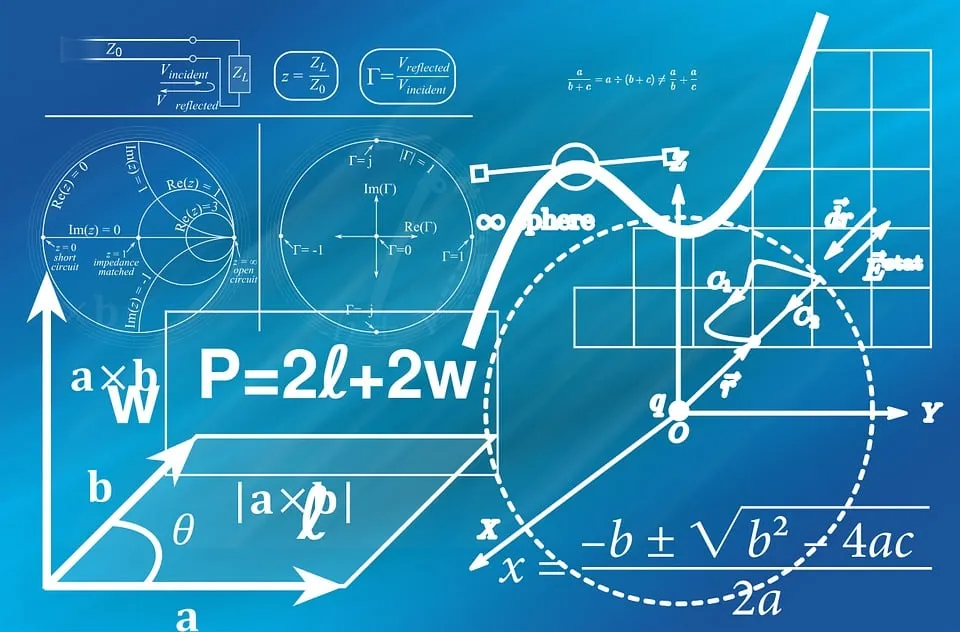Pixabay Image Source

## Topics

• Review Of FOIL For Expanding Two Binomials
• Review Of Square Roots
• Difference Of Squares Factoring Examples
• Simplifying Rational Expressions

## Review Of FOIL For Expanding Two Binomials

A binomial is a mathematical expression which contains two terms. Examples of binomials include: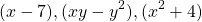When it comes to multiplying binomials, you do have to be careful in doing so. Some math students accidentally just multiply the first term of the first bracket with the second bracket or they do something strange.

Given two binomials such as `(a + b)` and `(c + d)`. Multiplying these two binomials together yields this product.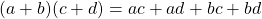The acronym FOIL helps here as:

• F is for First for `ac`
• O is for Outer which is for `ad` as `a` is the first in the left bracket and `d` is the second for the right bracket.
• I is for Inner for `bc`
• L is for Last for `bd` as `b` is the last term in the first bracket & `d` is the last term in the second bracket.

Example

Expand.

• First: x-squared times y
• Outer: x-squared time negative 7
• Inner: Negative 3 times y
• Last: Negative 3 times negative 7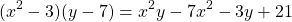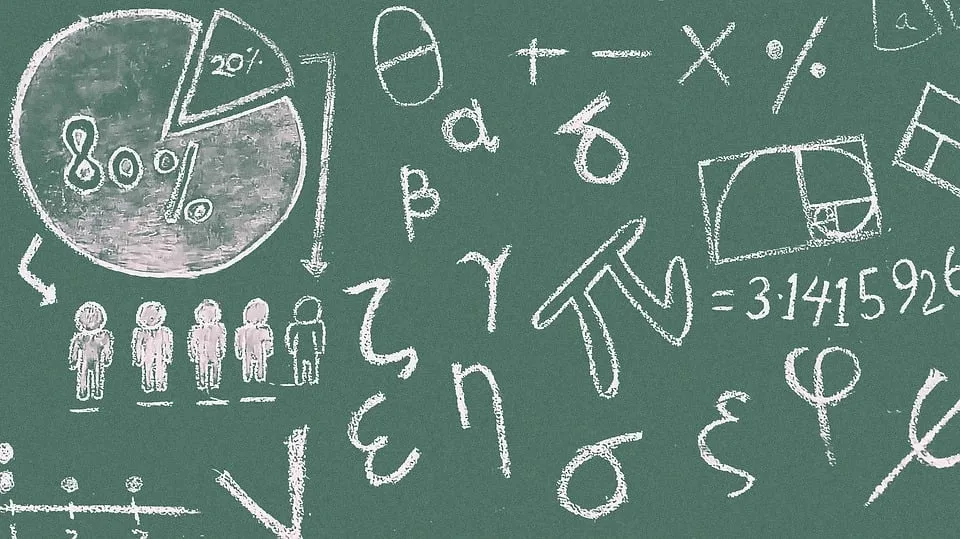Pixabay Image Source

## Review Of Square Roots

It is also a good idea to review square roots. The square root of a number is a number multiplied by itself to obtain the original number. Five is the square root of 25 as 5 times 5 is 25. Ten is the square root of 100 as 10 times 10 is 100.

We deal with whole numbers for square roots and perfect squares. Here is a sample chart.

Whole Number (Perfect Square)Square Root
11
42
93
164
255
366
497
648
819
10010
12111
14412

## Difference Of Squares Factoring Examples

The algebra technique of factoring is essentially the reverse of expanding binomials and the reverse of the distributive law. Factoring is useful for simplifying algebraic steps and for solving equations. It may not have direct real world applications but think of learning factoring as adding more tools to the math toolbox for calculations and problem solving.

Difference of squares factoring only works when there is a binomial expression. Both terms are perfect squares with the second term after a minus sign.

Example One

Factor.

We have two terms with a minus sign so this is good. The square root of 4 is 2. For x-squared the square root of that is just x. It is because `x` times `x` is x-squared. How we factor this is as follows.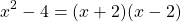The x-squared minus 4 is split into a product of two binomials. The square root of x-squared is x which appears in the beginning of both brackets. The square root of 4 is 2 but it is technically plus 2 and minus 2. This is why we see `(x + 2)` and `(x - 2)` to account for plus and minus 2 being the square root of 4.

The general formula for difference of squares factoring is: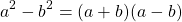Example Two

Having something like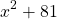is not valid for difference of squares factoring as we have a plus sign. You could do it if you want to deal with complex/imaginary numbers which is something high school students do not really touch.

Example Three

If you have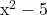, this does not work too well for difference of squares factoring. It is because 5 is not a perfect square and there is no whole number multiplied by itself to obtain 5.

In some cases, you may have to do difference of squares factoring if you need to simplify a fraction or something. You would have just the square root of 5 to deal with as an exact value.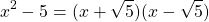Example Four - Not As Obvious Perfect Squares

Factor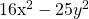.

These perfect squares are not as nice as the second term is algebraic instead of a nice number like 100. The square root of 16 x-squared is 4x. It is because 4x times 4x is 16 x-squared. For the 25 y-squared the square root of that is 5y. Take the square root of the number time the square root of the variable part.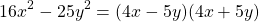Example Five - Multiple Difference of Squares Factoring

There are times when you have to apply difference of squares factoring more than once.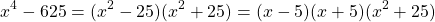For the second difference of squares factoring it is applied on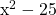.Pixabay Image Source

## Simplifying Rational Expressions

Factoring methods do come in handy when it comes to simplifying fractions with algebraic expressions.

Example One

Simplify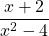.

This case is quite easy as we apply the difference of squares factoring method on the denominator.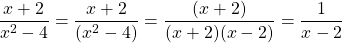Example Two

Simplify.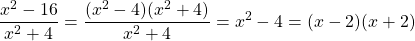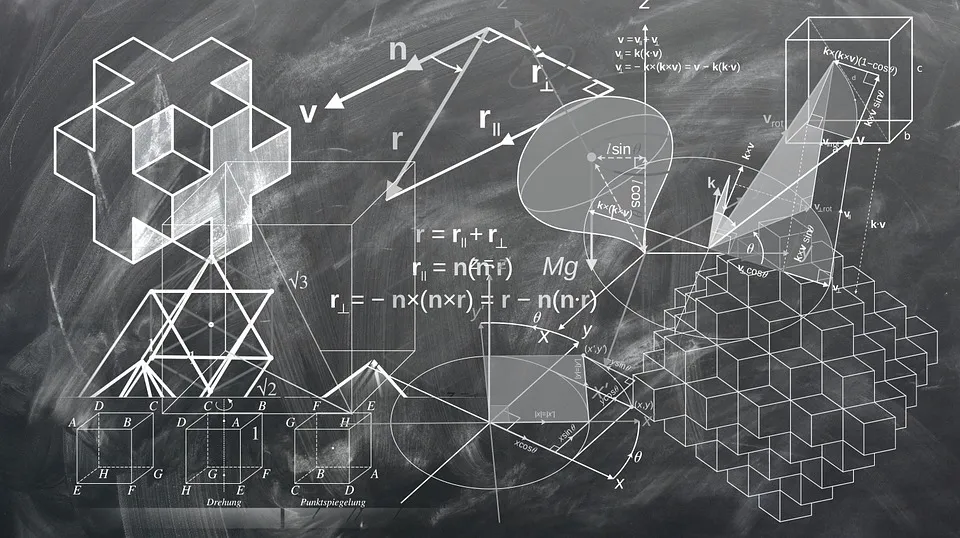Pixabay Image Source